# Bohm-Aharonov effect

(diff) ← Older revision | Latest revision (diff) | Newer revision → (diff)

A phenomenon in which the topological non-triviality of a gauge field is measurable physically [a1]. Moreover, this topological non-triviality, which can be expressed as a number, say, is a global topological invariant and so is not expressible by a local formula; this latter point being in contrast to a simpler topological invariant such as the dimension of the underlying space, which is deducible locally.

To demonstrate this effect physically, one arranges that a non-simply-connected regionof space has zero electromagnetic field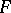. This electromagnetic fieldis related to the gauge fieldby the usual relation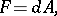where the usual differential form language has been used forandso that, if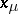are local coordinates on, one hasGiven thisand, one can devise an experiment in which one measures a diffraction pattern associated with the parallel transport, or holonomy, of the gauge fieldround a non-contractible loopin(cf. also Holonomy group). The action of parallel transport on multi-linear objects, viewed either as vectors, spinors, tensors, etc., or as sections of the appropriate bundles, is via the operator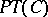, where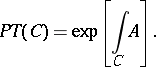Differential topology provides immediately the means to see thatis non-trivial. The argument goes as follows: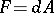so that the vanishing ofgivesHencedetermines a de Rham cohomology classand one has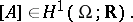It is clear from Stokes' theorem (cf. Stokes theorem) that the integral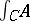only depends on the homotopy class of the loop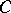. In addition, the loopdetermines a homology class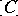, where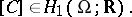This means that the integral(which can be taken equal to the number) is just the dual pairing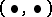between cohomology and homology, i.e.An experiment to test this for the electromagnetic field was done in [a2]. The experimental setup is of the Young's slits type, where electrons replace photons and with the addition of a very thin solenoid. The electrons pass through the slits and on either side of the solenoid an interference pattern is then detected. The interference pattern is first measured with the solenoid off. This pattern is then found to change when the solenoid is switched on, even though the electrons always pass through a region where the fieldis zero.

Mathematically speaking one realizes the solenoid by a cylinderso that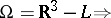The loopis the union of the electron paths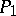and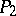.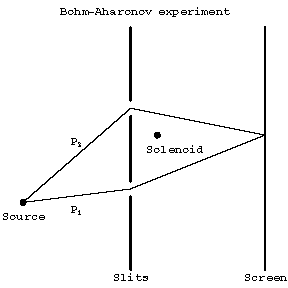Figure: b110690a

A schematic Bohm–Aharonov experiment

The Bohm–Aharonov effect shows that the gauge potentialis a more basic object than the electromagnetic field.

Geometrically speaking,is a connection andis a curvature. Hence, in purely geometrical language one can describe the situation by saying that a flat connection (i.e. one for which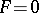, cf. Parallel displacement) need not be trivial ifis not simply connected, i.e. if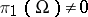. This non-triviality of flat connections whenextends to the non-Abelian or Yang–Mills case.

For a Yang–Mills-connection(withnon-Abelian), the curvatureis given byFurther, ifis defined on a bundle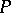over a manifoldand if it is assumed that, as in the Abelian case,then the integralonly depends on the homotopy class of, which is also denoted by(the context should prevent any confusion with homology classes). Adding to this the fact that the parallel transport operator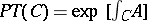has the property that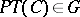, one can useto define the following linear mapping: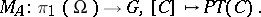One can check that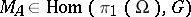so that, asvaries, the flat-connectionongives a representation of the fundamental groupin; it is easy to verify that if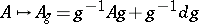under a gauge transformation, then thisacts onvia the adjoint action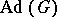. In other words, it acts by conjugation, i.e.,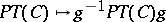. But this means that the representations defined by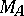andare equivalent, thus the gauge equivalence class of flat connections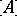is characterized by an element of the quotient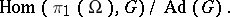Hence the moduli space of gauge-inequivalent flat connections is the spaceThe holonomy group elementis also of central interest elsewhere. It occurs in the study of the adiabatic periodic change of parameters of a quantum system described in quantum field theory, [a3], [a4], where it is called a Wilson line or Wilson loop, and in mathematics. One of the most important of these concerns the quantum field theory formulation of knot invariants [a5], such as the Jones polynomial [a6]. Flat connections also play a distinguished part in this theory.